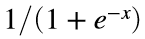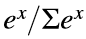# Problem 58882. Neural Nets: Activation functions

Return values of selected Activation function type for value,vector, and matrices.
y=Activation(x,id); where id is 1:4 for ReLU, sigmoid, hyperbolic_tan, Softmax
ReLU: Rectified Linear Unit, clips negatives max(0,x) Trains faster than sigmoid
Sigmoid: Exponential normalization [0:1]HyperTan: Normalization[-1:1] tanh(x)
Softmax: Normalizes output sum to 1, individual values [0:1]Used on Output node
Working though a series of Neural Net challenges from Perceptron, Hidden Layers, Back Propogation, ..., to the Convolutional Neural Net/Training for Handwritten Digits from Mnist.
Might take a day or two to completely cover Neural Nets in a Matlab centric fashion.
Essentially Out=Softmax(ReLU(X*W)*WP)

### Solution Stats

27.59% Correct | 72.41% Incorrect
Last Solution submitted on Oct 29, 2023

### Community Treasure Hunt

Find the treasures in MATLAB Central and discover how the community can help you!

Start Hunting!